Question

# A closely wound, circular coil with radius 2.60 cm has 800 tums. Part A What must...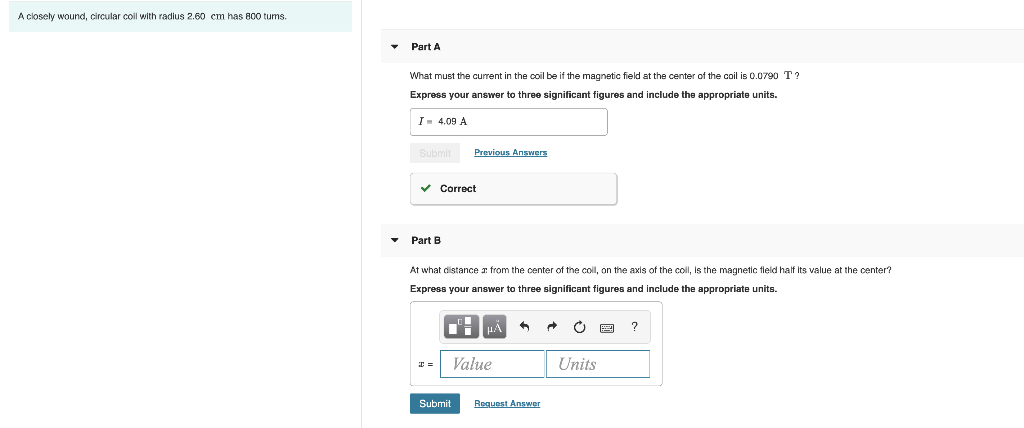A closely wound, circular coil with radius 2.60 cm has 800 tums. Part A What must the current in the coil be if the magnetic field at the center of the coil is 0.0790 T? Express your answer to three significant figures and include the appropriate units. I - 4.09 A Previous Answers ✓ Correct Part B At what distance from the center of the coil, on the axis of the coll, is the magnetic field half its value at the center? Express your answer to three significant figures and include the appropriate units. O O O ? I = Value Units Submit Request Answer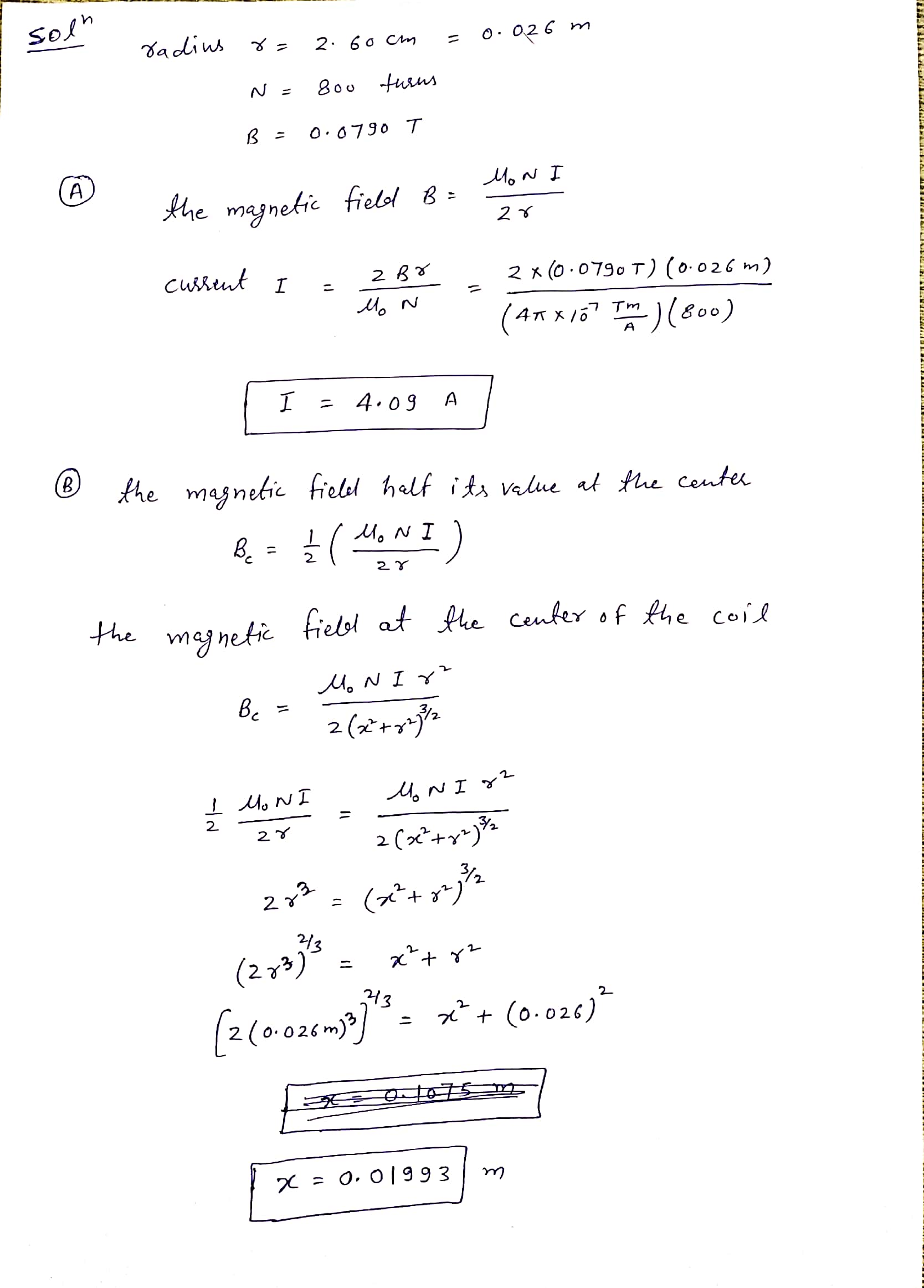#### Earn Coins

Coins can be redeemed for fabulous gifts.

Similar Homework Help Questions
• ### A closely wound, circular coil with radius 2.20 cm has 790 turns. Part A Part complete...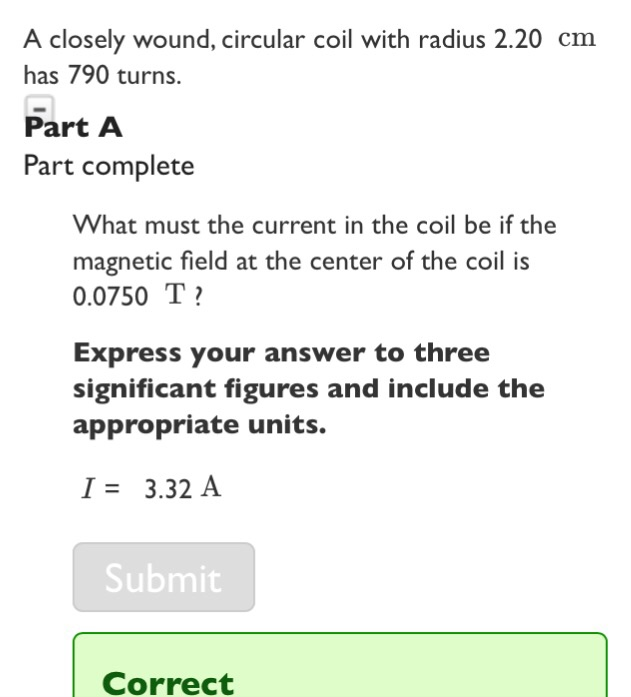A closely wound, circular coil with radius 2.20 cm has 790 turns. Part A Part complete What must the current in the coil be if the magnetic field at the center of the coil i:s 0.0750 T Express your answer to three significant figures and include the appropriate units. I3.32 A Submit Correct

• ### Exercise 28.34 - Enhanced - with Feedback A closely wound, circular coil with radius 2.30 cm...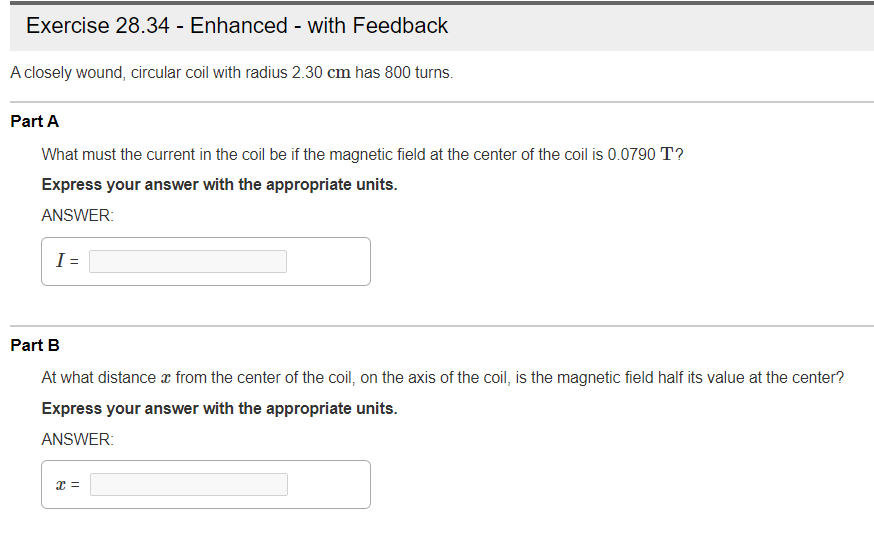Exercise 28.34 - Enhanced - with Feedback A closely wound, circular coil with radius 2.30 cm has 800 turns. Part A What must the current in the coil be if the magnetic field at the center of the coil is 0.0790 T? Express your answer with the appropriate units. ANSWER: I= Part B At what distance x from the center of the coil, on the axis of the coil, is the magnetic field half its value at the center? Express...

• ### Exercise 28.36 21 of 25 Part A What must the current in the coil be if...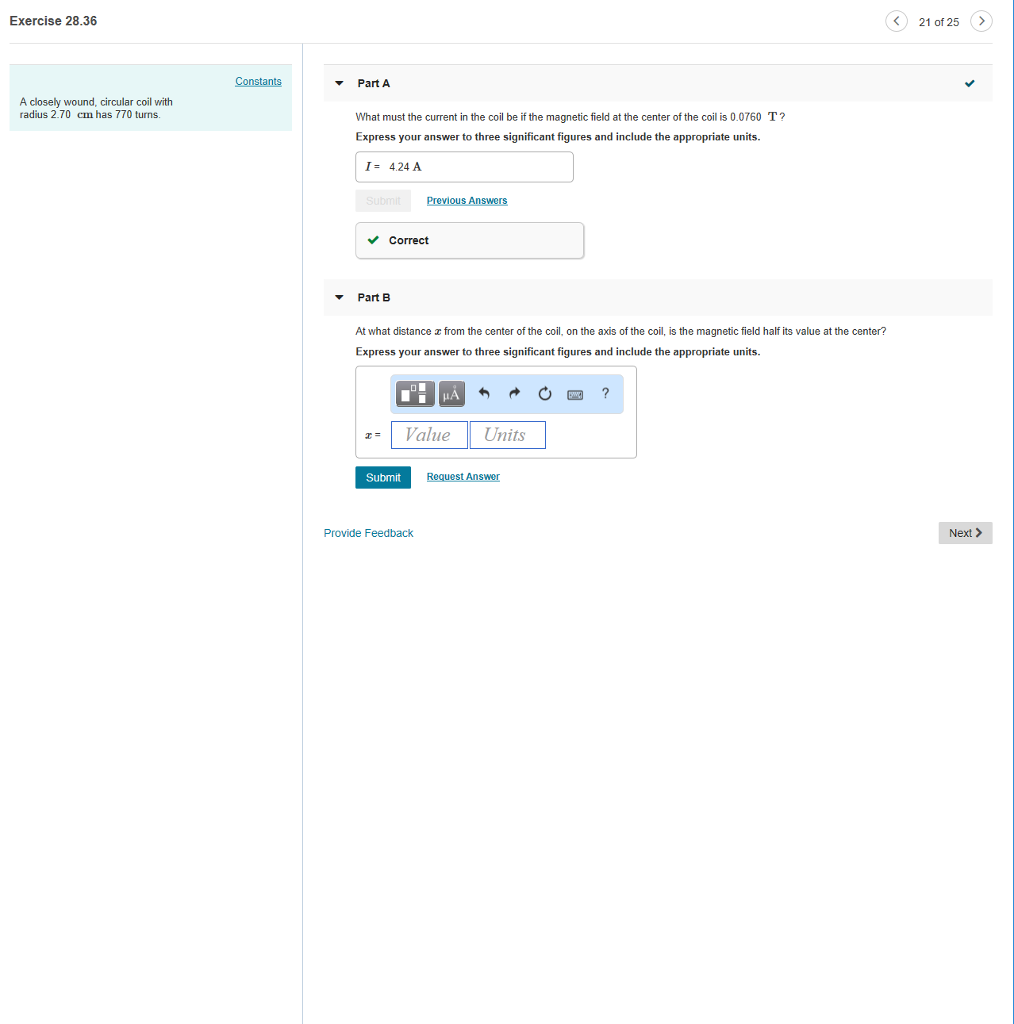Exercise 28.36 21 of 25 Part A What must the current in the coil be if the magnetic field at the center of the coil is 0.0760 T? Express your answer to three significant figures and include the appropriate units A closely wound, circular coil with radius 2.70 cm has 770 turns I-4.24 A Correct Part B At what distance x from the center of the coil, on the axis of the coil, is the magnetic field half its value...

• ### Reviel A closely wound, circular coil with a diameter of 4.00 cm has 690 turns and...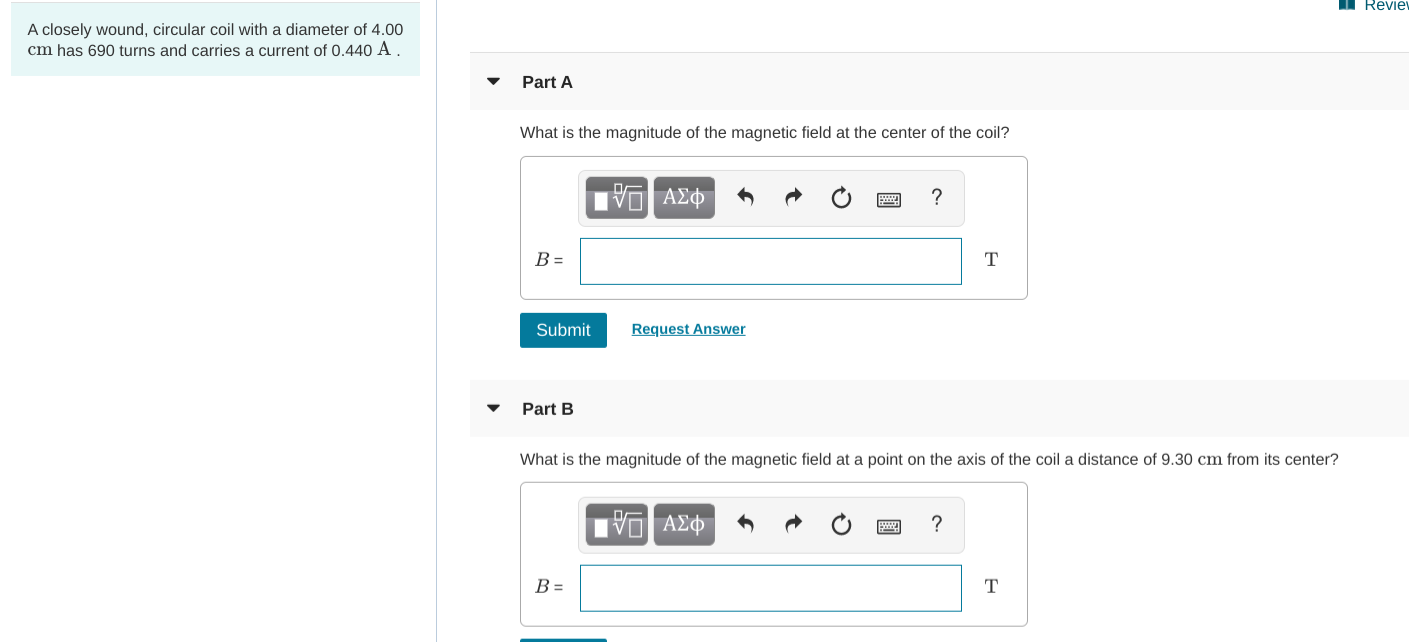Reviel A closely wound, circular coil with a diameter of 4.00 cm has 690 turns and carries a current of 0.440 A. Part A What is the magnitude of the magnetic field at the center of the coil? V AEO O ? B= Submit Request Answer Part B What is the magnitude of the magnetic field at a point on the axis of the coil a distance of 9.30 cm from its center? O AO O ?

• ### A closely wound circular coil with a diameter of 4.00 cm has 600 turns and carries...

A closely wound circular coil with a diameter of 4.00 cm has 600 turns and carries a current of 0.510 A . What is the magnetic field at the center of the coil?

• ### A closely wound circular coil with a diameter of 4.10 cm has 520 turns and carries...

A closely wound circular coil with a diameter of 4.10 cm has 520 turns and carries a current of 0.420 A. (a) What is the magnitude of the magnetic field at the center of the coil? T (b) What is the magnitude of the magnetic field at a point on the axis of the coil 8.00 cm from its center? T

• ### A closely wound circular coil with a diameter of 4.00cm has 600 turns and carries a current of...

A closely wound circular coil with a diameter of 4.00cm has 600 turns and carries a current of 0.500 A. What is the magnetic field at the center of the coil?

• ### Part B A circular loop in the plane of a paper lies in a 0.45 T...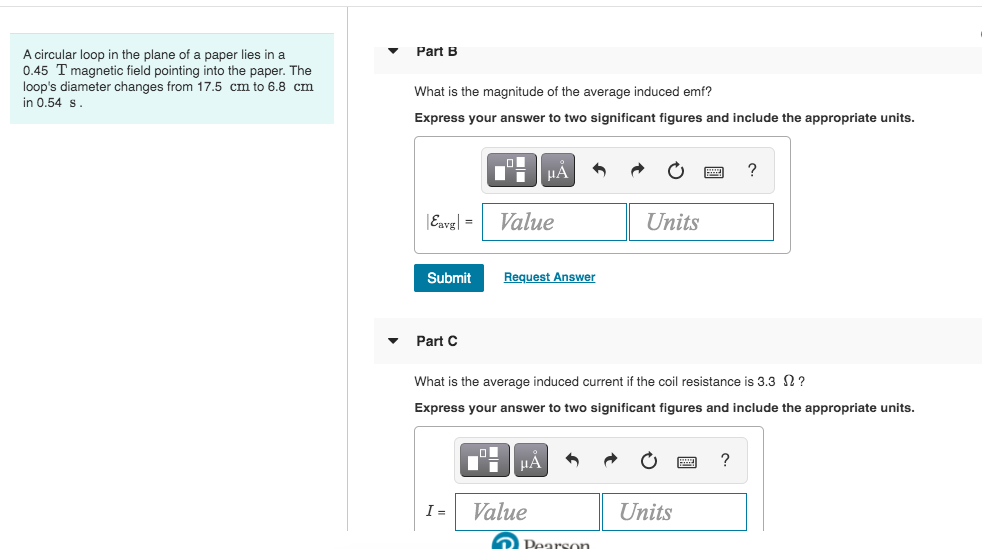Part B A circular loop in the plane of a paper lies in a 0.45 T magnetic field pointing into the paper. The loop's diameter changes from 17.5 cm to 6.8 cm in 0.54 s What is the magnitude of the average induced emf? Express your answer to two significant figures and include the appropriate units. UA ? Eavg) = Value Units Submit Request Answer Part C What is the average induced current if the coil resistance is 3.3 12?...

• ### A circular coil that has 120 turns and a radius of 10.0 cm lies in a...

A circular coil that has 120 turns and a radius of 10.0 cm lies in a magnetic field that has a magnitude of 0.0650 T directed perpendicular to the coil. What is the magnetic flux through the coil? (Express your answer to three significant figures.) The magnetic field through the coil is increased steadily to 0.100 T over a time interval of 0.300 s. What is the magnitude of the emf induced in the coil during the time interval? (Express...

• ### Constants A 11-cm-diameter circular loop of wire is placed in a 1.37-T magnetic field. Part A...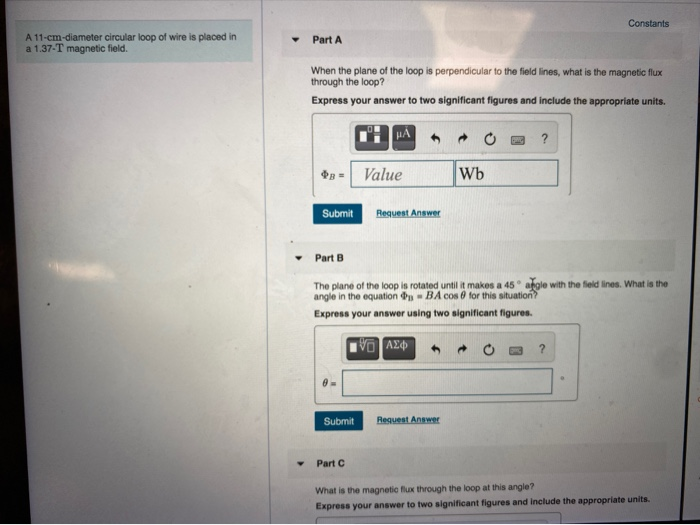Constants A 11-cm-diameter circular loop of wire is placed in a 1.37-T magnetic field. Part A When the plane of the loop is perpendicular to the field lines, what is the magnetic flux through the loop? Express your answer to two significant figures and include the appropriate units. NA ? Value Wb Submit Request Answer Part B The plane of the loop is rotated until it makes a 45 agle with the field lines. What is the angle in the...